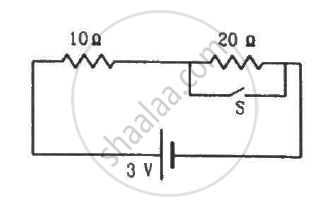Department of Pre-University Education, KarnatakaPUC Karnataka Science Class 12
Advertisement Remove all ads

# Consider the Circuit Shown in the Figure. Find the Current Through the 10 ω Resistor When the Switch S Is (A) Open (B) Closed. - Physics

Sum

Consider the circuit shown in the figure. Find the current through the 10 Ω resistor when the switch S is (a) open (b) closed.Advertisement Remove all ads

#### Solution

(a) When S is open, the resistors will be connected in series, as the current will flow through the 20 Ω resistor. The effective resistance,

$R_{eff} = \left( 10 + 20 \right) \Omega = 30 \Omega$

The current flowing through the circuit,

$i = \left( \frac{3}{30} \right) A = 0 . 1 A$

(b) When S is closed, current will flow through the switch that offers the least resistive path. So, the 20 Ω resistance will be ineffective in this case. The effective resistance in this case,

$R_{eff} = 10 \Omega$

The current flowing through the circuit,

$i = \left( \frac{3}{10} \right) A = 0 . 3 A$

Is there an error in this question or solution?
Advertisement Remove all ads

#### APPEARS IN

HC Verma Class 11, Class 12 Concepts of Physics Vol. 2
Chapter 10 Electric Current in Conductors
Q 32 | Page 199
Advertisement Remove all ads

#### Video TutorialsVIEW ALL 

Advertisement Remove all ads
Share
Notifications

View all notifications

Forgot password?